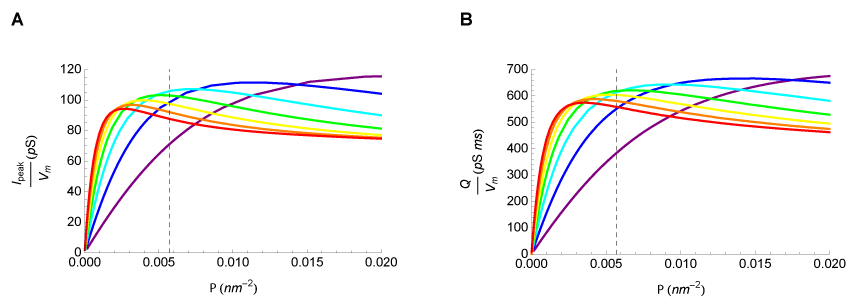Figure 7: Receptor-limited diffusion case: Effects of receptor density on peak current and total charge. Peak current (A) and total charge (B) were calculated as a function of receptor density. The graphs illustrate the relationship for the following radial dimensions: rPSD=42 nm (purple), rPSD=62 nm (blue), rPSD=82 nm (cyan), rPSD=102 nm (green), rPSD=122 nm (yellow), rPSD=142 nm (orange) and rPSD=162 nm (red). The dashed line corresponds to the maximal receptor density for the AMPA receptor.